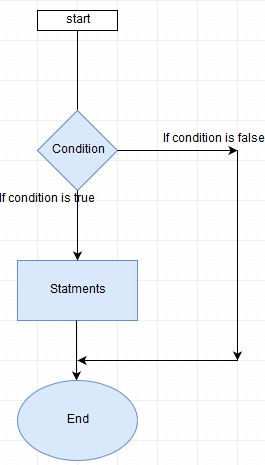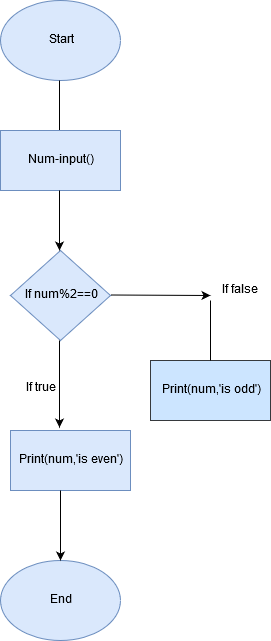### Unit 1 - Conditional Statements and Simple Programs

CBSE Revision Notes

Class-11 Computer Science (New Syllabus)
Unit 1: Programming and Computational Thinking (PCT-1)

Conditional Statements and Simple Programs

Conditional statements

In conditional statement we check for specific conditions. its value can be either true or false. If it’s true some statements are executed else some other statements will be executed. Conditions can be checked using some operators like

< (less than), <=(less than equal to), >(greater than), >=(greater than equal to), ==(equal to), !=(not equal to). Let’s see how to use these operators in the expression and what will be the output of each expression.

 20<40 true 40>80 False x<=10 True if value of x is less than or equal to 10 else false x!=y True if x is not equal to y else false x==y True if value of x and y is same else false 20<=20 True

Let’s see with the help of flowchart how it actually works.There are 3 ways to check conditions. if, if-else, if–elif-else. If condition is true

if: if checks condition if it’s true it will execute the block of statements within if the block. If condition is false it will skip that block. Syntax of if block is as follows

If condition:
block

let’s understand this with an example.

print('Enter number')
num=eval(input())
if num%2==0:
print(num,' is even')

in the above program we will check if the number is even. First we are taking input from user here eval checks type of data it’s if its integer it will convert of text to integer. When we use input() function it treats everything as string in case of number we need to convert that into integer eval does it for us. In if block first we check the condition if the remainder of number is 0 then it’s even. If number is even it will execute the if block else it won’t do anything.

if-else: Unlike if it has 2 blocks if and else. If “if” block is true then it will execute that block if “if” block is false then it will execute else block. Syntax of if-else is as follows

If condition:

If_block

else:

else_block

Let’s understand this with an example

In this program we’ll check if number is even or odd

print('Enter number')

num=eval(input())

if num%2==0:

print(num,' is even')

else

print(num,’ is odd’)

in this example we are taking input from user then we are checking if remainder of that number is 0 then number is even else number is odd. Let’s now understand this with flowchart.if-elif-else: if-else can check at max 2 conditions one for true and other false but if we want to check more than 2 condition we can use if-elif-else. Syntax of if-elif-else

If condition:
if_block
elif condition:
elif_block
elif condition:
elif block
else:
else block

Let’s see an example where we’ll check which number we have entered

print(' Enter number')

num=eval(input())

if num<0:

print("Number less than 0")

elif num==0:

print("you've entered zero")

elif num==1:

print("you've entered one")

elif num==2:

print("you've entered Two")

elif num==3:

print("you've entered three")

elif num==4:

print("you've entered four")

elif num==5:

print("You've entered five")

else:

print("Number greater than 5")

this program will check if number is between 5 or greater than 5. E.g. we will enter 3 it will show number is 3. If we’ll enter 6 it will display number greater than 5.

Greater among 3 numbers:

print("Enter first number")

num1=eval(input())

print("Enter second number")

num2=eval(input())

print("Enter third number")

num3=eval(input())

if num1>num2:

if num1>num3:

print(num1,' is greater')

elif num2>num3:

print(num2,' is greater')

else:

print(num3,' is greater')

in above program ist we are checking is num1 is greater than num2 if it’s true then it’ll check num1 is greater than num3 if it’s also true then it’ll print num1 is greater . If num1 is not greater than num2 then elif condition will be checked if num2 is greater than num3 than it will print num2 is greater else it will print num3 is greater.